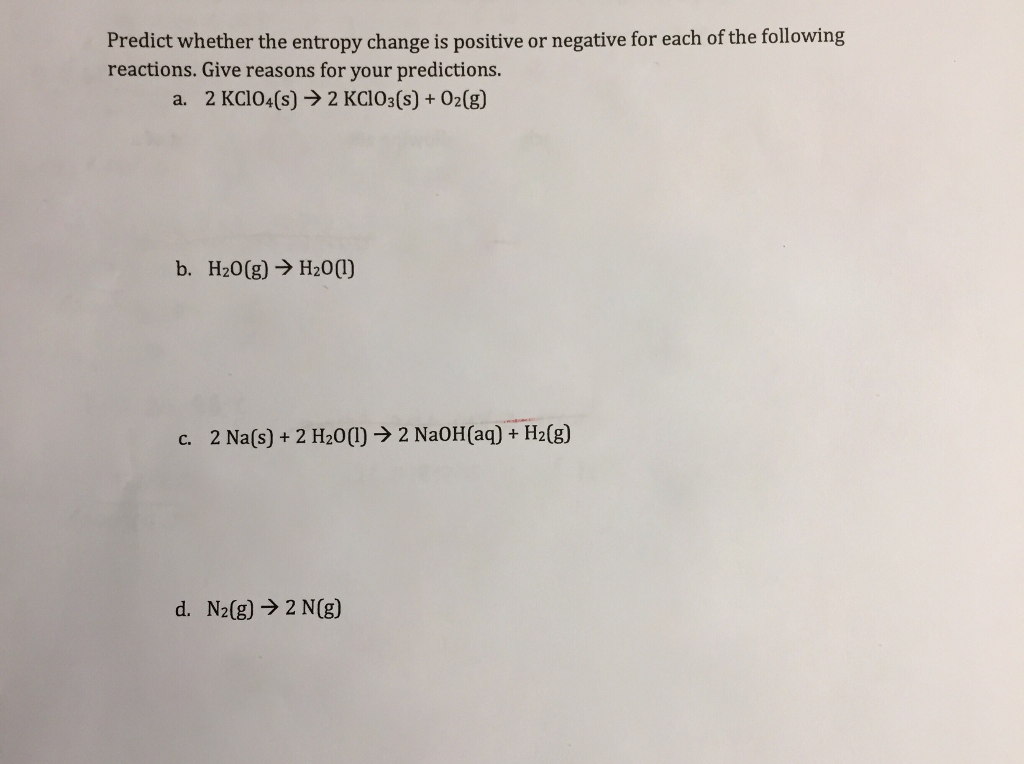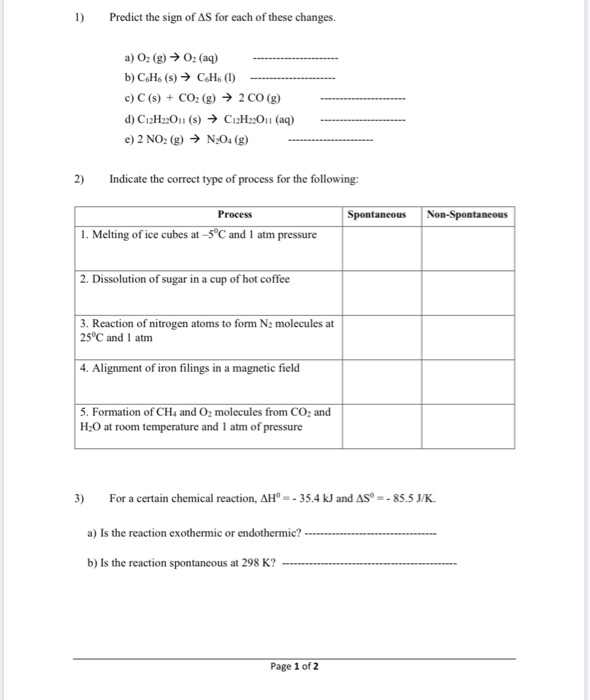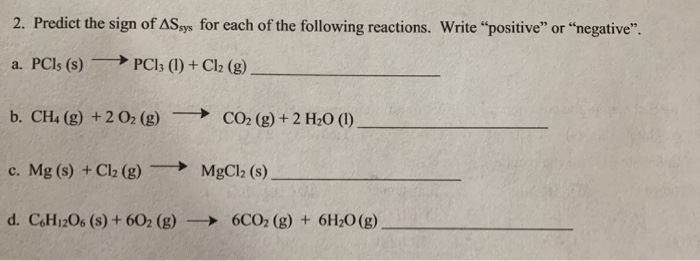Question

# Predict the sign of ΔS for each of the following; explain your reasoning O2(g) → O2(l)...

Predict the sign of ΔS for each of the following; explain your reasoning

O2(g) → O2(l)

2 KClO4(s) → 2 KClO3(s) + O2(g)

CH4(g) + 2 O2(g) → CO2(g) + 2 H2O(l)

Entropy is the degree of disorderness Or randomness is called entropy

DeltaS=products entropy-reactants entropy

Here oxygen gas is converted to liquid so the entropy is decreased

So DeltaS<0 (Negative)

Here 2 moles of solid is converted to 2 moles of solid+one mole of gas

So the entropy is increased

So deltaS is positive

C.

3 moles of gas is converted to one mole of gas + 2 moles of liquid

So deltaS is negative

#### Earn Coins

Coins can be redeemed for fabulous gifts.

Similar Homework Help Questions
• ### Predict the sign of the entropy change, ΔS∘, for each of the reaction displayed. Drag the appropriate items to their r...

Predict the sign of the entropy change, ΔS∘, for each of the reaction displayed. Drag the appropriate items to their respective bins. Pb2+(aq)+2Cl−(aq)→PbCl2(s) CaCO3(s)→CaO(s)+CO2(g) 2NH3(g)→N2(g)+3H2(g) 2Mg(s)+O2(g)→2MgO(s) C5H12(g)+8O2(g)→5CO2(g)+6H2O(g) H2O(l)→H2O(g) Positive                                                   Negative

• ### For each of the following reactions, predict the sign of ΔS°. 2Na(s) + Cl2(g) → 2NaCl(s)...

For each of the following reactions, predict the sign of ΔS°. 2Na(s) + Cl2(g) → 2NaCl(s) KBr(s) → K+(aq) + Br−(aq) 2H2(g) + O2(g) → 2H2O(l) C2H2(g) + 4F2(g) → 2CF4(g) + H2(g)

• ### Part A Predict the sign of the entropy change, ΔS∘, for each of the reaction displayed. Drag the appropriate items to...

Part APredict the sign of the entropy change, ΔS∘, for each of the reaction displayed.Drag the appropriate items to their respective bins.Ag+(aq)+Cl−(aq)→AgCl(s)2KClO3(s)→2KCl(s)+3O2(g)2N2O(g)→2N2(g)+O2(g)2Mg(s)+O2(g)→2MgO(s)C7H16(g)+11O2(g)→7CO2(g)+8H2O(g)H2O(l)→H2O(g)Part BCalculate the standard entropy change for the reaction2Mg(s)+O2(g)→2MgO(s)using the data from the following table:SubstanceΔH∘f (kJ/mol)ΔG∘f (kJ/mol)S∘ [J/(K⋅mol)]Mg(s)0.000.0032.70O2(g)0.000.00205.0MgO(s)-602.0-569.627.00Express your answer to four significant figures and include the appropriate units.ΔS∘ =

• ### The balanced chemical equation below shows the combustion of methanol: CH3OH(l) + 3O2(g) → 2 H2O(g)...

The balanced chemical equation below shows the combustion of methanol: CH3OH(l) + 3O2(g) → 2 H2O(g) + CO2(g) a) Predict the sign of ΔS for this reaction and briefly explain your reasoning b) Predict the sign of ΔH for this reaction and briefly explain your reasoning c) Is the sign of ΔG temperature dependent in this reaction? Briefly explain your reasoning

• ### Predict whether the entropy change is positive or negative for each of the following reactions. Give...Predict whether the entropy change is positive or negative for each of the following reactions. Give reasons for your predictions. a. 2 KClO4(s) + 2 KClO3(s) + O2(g) b. H2O(g) → H20(1) c. 2 Na(s) + 2 H20(1) ► 2 NaOH(aq) + H2(g) d. N2(g) → 2 N(g)

• ### Select all of the following that you would predict to have a negative ΔS. a) 2...

Select all of the following that you would predict to have a negative ΔS. a) 2 NO2 (g) → N2 (g) + 2 O2 (g) b) 2SO2 (g) + O2 (g) → 2 SO3 (g) c) 2 C6H6 (l) + 15 O2 (g) → 12 CO2 (g) + 6 H2O (g) d) 4 NO (g) + 6 H2O (g) → 4 NH3 (g) + 5 O2 (g) e) 2 BF3 (g) + 3 CaO (s) → B2O3 (s) + 3...

• ### Predict the sign of AS for each of these changes. a) O2 (g) → O2 (aq)...Predict the sign of AS for each of these changes. a) O2 (g) → O2 (aq) b) C.H. (8) → C.H. (1) - c) C(s) + CO2(g) → 2 CO (g) d) C12H22011 (8) ► 12H2011 (aq) e) 2 NO2(g) → N.04 (g) 2) Indicate the correct type of process for the following: Process Spontaneous Non-Spontaneous 1. Melting of ice cubes at -5°C and 1 atm pressure 2. Dissolution of sugar in a cup of hot coffee 3. Reaction of...

• ### 2. Predict the sign of ASsys for each of the following reactions. Write "positive" or "negative"....2. Predict the sign of ASsys for each of the following reactions. Write "positive" or "negative". a. PСls (s) → PCI; (1) + Cl2 (g) b. CH4 (g) + 2 O2 (g) → CO2 (g) + 2 H20 (1) c. Mg(s) + Cl2 (g) MgCl2 (s). d. CH1206 (8) + 602 (g) 6CO2 (g) + 6H20 (g)

• ### Calculate the standard-state entropy for the following reaction: 1 CH4(g) + 2 O2(g) ⟶ 1 CO2(g) + 2 H2O(l) (If applica...

Calculate the standard-state entropy for the following reaction: 1 CH4(g) + 2 O2(g) ⟶ 1 CO2(g) + 2 H2O(l) (If applicable, coefficients of one have been included for clarity.) The standard entropy values are given in the table. Formula S∘ J/(K⋅mol) CO2(g) 214 H2O(l) 189 CH4(g) 186 O2(g) 205

• ### Which of the following species acts as a reducing agent during the following reaction? CH4(g)+O2(g)⟶CO2(g)+H2O(l) Options:...

Which of the following species acts as a reducing agent during the following reaction? CH4(g)+O2(g)⟶CO2(g)+H2O(l) Options: (a) CH4 (b) O2 (c) CO2 (d) H2O